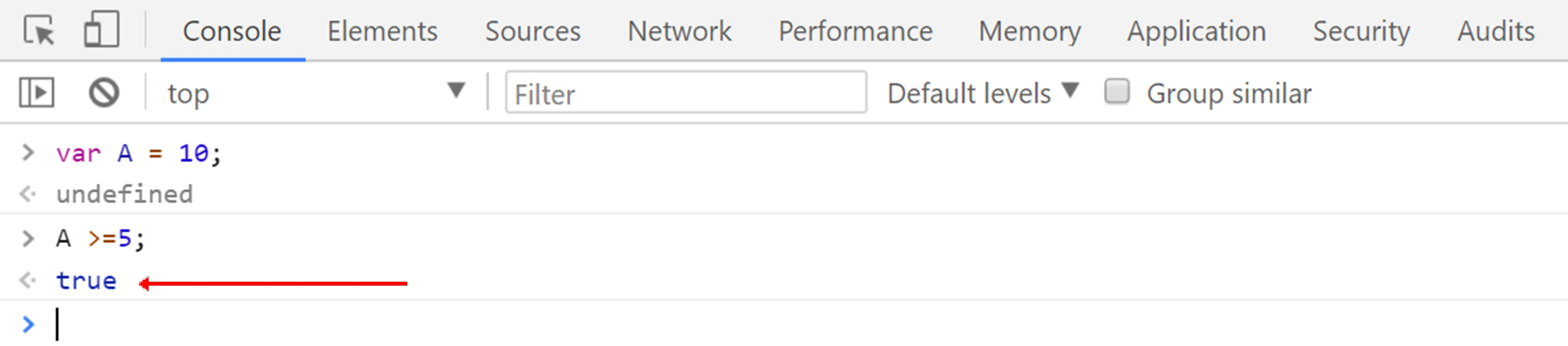# Lesson 7 Boolean Logic in JavaScript

In this lesson, we are learning Boolean logic, and we will also discuss an important topic which is how we make decisions in our JavaScript code.
We will also learn comparison operators.

In the previous lesson we have given a short overview of Boolean logic when we were covering data types in JavaScript, so Booleans are very simple.
There are two possible options for Boolean value:

• They are True or Yes
• They are False or No
So Boolean logic is simply writing a statement to evaluate the idea that the statement is either True or False and eventually, we will use this statement to actually run certain parts of our code if something is true and some other part of our code if something else is false

So we use these kinds of statement to evaluate something like if a person X logged in to some Website, that’s true or false or a person X has more than \$15,000 balance in their bank account, that’s true or false.

The first thing we will start with is comparison operators and comparison operation are things that most of us use all the time in our daily life. We use the comparison operator to compare two values to one another and it is how JavaScript does the same thing.

Here is the chart below where we have using all the comparison operators.

## Comparison Operators

### Assuming A = 10

Operator Name Example Result
> Greater than A > 20 False
>= Greater than or equal to A >= 10 True
< Less than A < -50 False
<= Less than or equal to A <= 100 True
== Equal to A == "10" True
!= Not equal to A != "b" True
=== Equal value and type A === "10" False
!== Not equal value or equal type A !== "10" True

If you see the operator in above chart where it depicts that is A >20? Obviously, A’s value is 10 can’t be equal to 20, so definitely it evaluates the value to be false.

So here is another simple example, let suppose there is a variable A whose value is 10
A = 10
But when we comparing value of A to number 5 and assuming A is greater than and equal to 5.
We use ‘greater than and equal to sign’ >= when we are not sure about the exact value.
A >= 5
So it evaluate the value to be true.
Here is example of JavaScript code below, where we have declared a variable A and assign it value 10.
We then comparing variable A to 5 number and saying value of A which is 10 is greater than and equal to 5 and it evaluate the output as a true.In this lesson, we have learned Boolean logic, and we have also discussed an important topic which is how we make decisions in our JavaScript code.
We have learned comparison operators with some example.